## Revision Notes of Ch 6 Lines and Angles Class 9th Math

Topics in the Chapter

• Corresponding angles
• Corresponding angles axiom
• Converse of corresponding angles axiom
• Alternate angles
• Alternate angles axiom
• Converse of alternate angles axiom
• Property of interior angles on the same side of a transversal
• Converse of the property of interior angles on the same side of a transversal
• Angle sum property of triangles
• Relation between the vertex angle and the angles made by the bisectors of the remaining angles

Corresponding anglesCorresponding angles axiom

If a transversal intersects two parallel lines, then each pair of corresponding angles is equal.
In the above figure, if lines l and m become parallel then we will have following pair of equal angles:
∠1 =∠5, ∠2 =∠6, ∠3 =∠7 and ∠4 = ∠8

Converse of corresponding angles axiom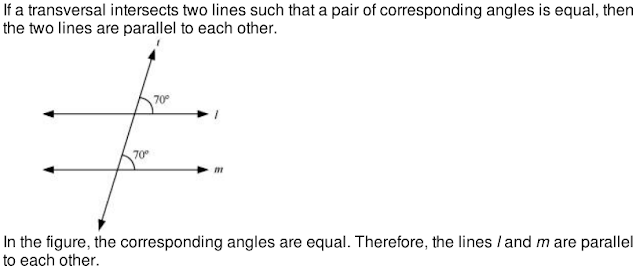Alternate angles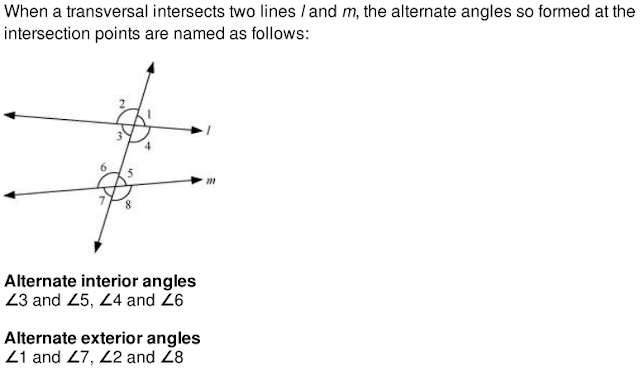Alternate angles axiomConverse of alternate angles axiom

If a transversal intersects two lines such that the angles in a pair of alternate angles are equal, then the two lines are parallel.Property of interior angles on the same side of a transversalConverse of the property of interior angles on the same side of a transversal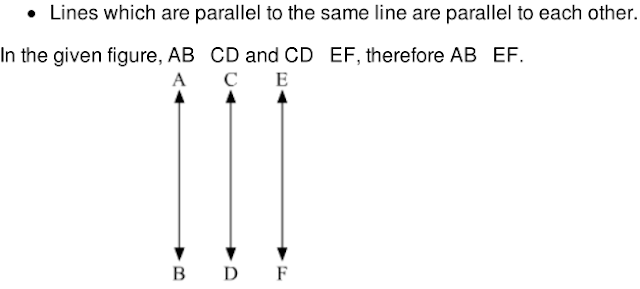In the given figure, line AB is parallel to CD and CD is parallel to EF. Find the value of xIt is given that AB || CD and CD || EF.
We know that the lines which are parallel to the same line are parallel to each other.
Therefore, AB || EF.
⇒ x = 75° (Alternate interior angles)

Angle sum property of triangles

The sum of all the three interior angles of a triangle is 180°.
∴ ∠A + ∠B + ∠C = 180°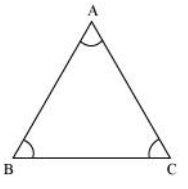Example:If the measures of the angles of a triangle are in the ratio 2: 4: 6, then find all the angles of the triangle.

Solution:Ratio of the measures of angles = 2: 4: 6
Therefore, let the angles of the triangle measure 2x, 4x, and 6x.
Now, 2x + 4x + 6x = 180° {By angle sum property of triangles}
⇒ 12x = 180°
⇒ x = 15°
Thus, the angles of the triangle are
2x =2 × 15° = 30°,
4x = 3 × 15° = 60°
6x = 6 × 15° = 90°.
The measure of one of angle is 90°.

Facts deduced from angle sum property of triangles

• There can be no triangle with two right angles or two obtuse angles.
• There can be no triangle with all angles less than or greater than 60°.

Relation between the vertex angle and the angles made by the bisectors of the remaining angles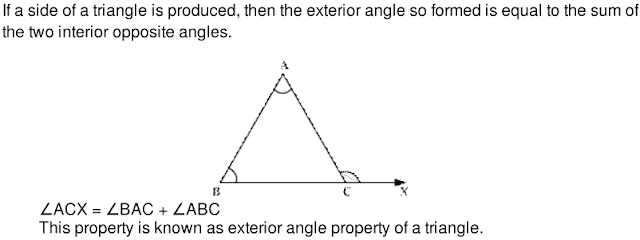Find the value of x in the following figure.∠QRS is an exterior angle of ΔPQR. It is thus equal to the sum of its interior opposite angles.
∴ ∠QRS = ∠QPR + ∠PQR
⇒ x = 65° + 70° = 135°
Thus, the value of x is 135°.
Two exterior angles can be drawn at each vertex of triangle. The two angles thus drawn have an equal measure and are equal to the sum of the two opposite interior angles.